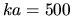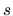## Geometrical optics approximation (GO)

For particles of very large sizes, scattering can be considered as a result of interference of the rays having multiple reflections and refractions according to the laws of geometrical optics. Diffraction at the particle edges which gives a sharp maximum in the region of small angles can be taken into account separately. The formulation of general principles, computational schemes and discussion of obtained results within the GO can be found in books [7,11,124]. The papers [413,414] presented a method where the scattering field was expressed via the field at the particle surface using the Huygens-Kirchhoff principle, and reflection and refraction laws were employed to find the unknown field (the approach was called physical optics approximation). Utilizing the GO method the author of  solved the general question why the mean extinction cross-section for an ensemble of large non-spherical particles is always larger than this cross-section for equivolume spheres. Illustrations of various applications of the method are given in [28,30,155,192,263,412,425,427]. Note also a recent paper  where the GO method is applied to analysis of light scattering by spheres with the size parameterand the discovered theoretical and experimental relationships in the oscillation ofandcomponents of intensity were used for successful solution of the inverse problem of sizing. The accuracy of the GO method for non-spherical particles was studied in a recent paper  by comparison with the exact T-matrix method results.To the top
Created by V.I.